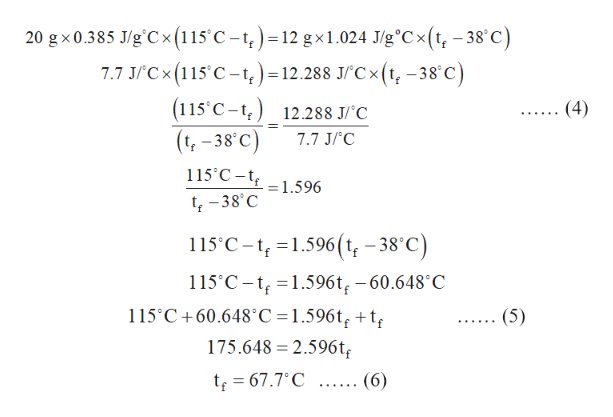# A 20-gram piece of copper (c = 0.385 J/g degrees celsius) at 115 degrees Celsius is placed in contact with a 12-gram sample of magnesium (c = 1.024 J/g degrees celsius) at 38 degrees celsius. What will the final temperature of the two metals be when they reach an equilibrium condition?

Question
6 views

A 20-gram piece of copper (c = 0.385 J/g degrees celsius) at 115 degrees Celsius is placed in contact with a 12-gram sample of magnesium (c = 1.024 J/g degrees celsius) at 38 degrees celsius. What will the final temperature of the two metals be when they reach an equilibrium condition?

check_circle

Step 1

The formula for specific heat can be written below in which q represents the heat energy in Joules, m represents the mass of the substance in kilogram, c represents the specific heat and ∆t represents the change in the temperature.

q = mc∆t                                     …… (1)

The amount of heat lost by the copper metal(qc) is equal to amount of heat gained by sample of magnesium(qm). Thus, qc=qm. This is shown in equation (2) in which mc is the mass of copper, cc is the specific heat for copper, ∆tc is the change in temperature of the copper, mm is the mass of magnesium , cm is the specific heat for magnesium and  ∆tis the change in temperature of the magnesium.

mccc∆tc= mmcm∆tm                       …… (2)

mccc (ti -tf )= mmcm(tf -ti )             …… (3)

Step 2

Substitute 20 g for mc, 115○C for ∆ti, 12 g for mm, 1.024 J/g○C for cm and 38 ○C for ∆ti in equation (3) as shown in equation (4).

Further, solve the above e...help_outlineImage Transcriptionclose20 g x 0.385 J/g'Cx (115'C -t)=12 gx1.024 J/g°Cx(t, -38°'c) Cx (115'C-t,)=12.288 JC(t, -38 C) (115 C-t 12.288 J/C (t-38 C) (4) 7.7 J/'C 115 C-t = 1.596 t-38 C 115 C-1.596(t,-38°C) 115 C-1.596t -60.648 C 115C+60.648°C = 1.596t, +t (5) 175.648 2.596t t 67.7'C .... (6) fullscreen

### Want to see the full answer?

See Solution

#### Want to see this answer and more?

Solutions are written by subject experts who are available 24/7. Questions are typically answered within 1 hour.*

See Solution
*Response times may vary by subject and question.
Tagged in

### Chemistry